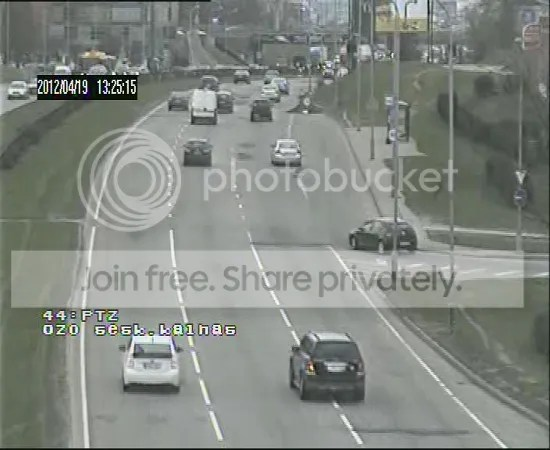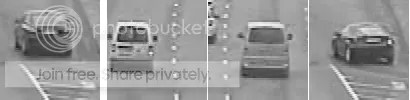Want to share your content on R-bloggers? click here if you have a blog, or here if you don't.

There are plenty of data on internet, however it is raw data. Think for a second about public surveillance cameras – useful to check the traffic on the route or busy place, but anything else? What if you want to know how many cars are on the route? How many car were yesterday at the same time? Given so many cars on the route, how much polluted air in the area?
While working on the road map for data dive event, I started to wonder, how feasible is to use data of public surveillance cameras. So I quickly built a pilot project and now I would like to share my experience.

First step – data acquisition. At beginning I was thinking to plug my smartphone somewhere and collect data of the busy route.  Nevertheless, I quickly found surveillance cameras in Vilnius and started to collect images. Run a search and I’m sure, that you will find them in your city:Here is bash script, which I use to collect images:

 ```#you need full path for crontab cd /home/git/carCount/img a=`date +%s` b=\${a}_4.jpg wget -O \$b -q "http://www.sviesoforai.lt/map/camera.aspx?size=full&image=K7742-1.jpg&rnd=0.15417794161476195"```

Data preparation. After while you will have enough data to train your machine (for beginning more than 30 images should be O.K.).
How do we train the algorithm? The goal is to identify the cars in a given image. That means, that we have to provide the examples of positive images (clear image of the cars) and negative images (no car, parts of the car and etc.). Important note – we don’t feed whole image, but we cut a chosen image with sliding window (100×100 in my case). 4 examples of positive images:Meanwhile, it is worth converting each image to portable grey format PGM. For this specific task, we can sacrifice information about the color of the car – it won’t improve prediction. Besides, PGM images can be loaded into R and easily transformed into matrix. Here is bash script, which converts jpg to pgm and slices each image:

 ```#remove image duplicates find . -maxdepth 1 -type f -exec md5sum {} \; >test.txt awk 'a[\$1]++ {gsub(/^\*/,"",\$2); print "rm ", \$2}' test.txt |sh rm test.txt   #convert jpg   if [ -d "out" ]; then rm -r out fi mkdir out for k in \$(ls *.jpg); do convert \$k out/\$k.pgm; done   cd out mkdir slide for filename in \$(ls *.pgm); do   w=`convert \$filename -print "%w" /dev/null` h=`convert \$filename -print "%h" /dev/null` let "ww= \$w/100" let "hh= \$h/100" for((y=150;y<=250;y+=50)) do for((i=100;i<=400;i+=50)) do echo "slide/\$i.\$filename" let "h_slide=\$i" convert \$filename -crop 100x100+\$i+\$y slide/\$y.\$i.\$filename done done done```

Training, predicting, cross validation. Now is time to open R, load 100×100 images from “train/out/slide” directory and train the algorithm. Important note – each image is a matrix, however you have to feed a matrix of all images to learning algorithm (support vector machine in my case). What you have to do is to “unroll” each image matrix into a vector, get 1X10000 vector and build a new matrix, where each row is an image.
Once training is done, load unseen data from “crossval/out/slide” directory and check “result/” directory, where you will find  images of the cars. R script, which does all above:

?View Code RSPLUS
 ```setwd('/home/git/carCount/')   ######read positives############ files=list.files('test/pos/') pos=matrix(nrow=NROW(files),ncol=100*100)   for(i in 1:NROW(files)) {   gray_file=read.pnm(paste('test/pos/',files[i],sep=''))   pos[i,]=c(gray_file@grey) } outcome=vector(length=NROW(files)) outcome[which(outcome!=1)]=1   ########read negatives############# files=list.files('test/neg/') neg=matrix(nrow=NROW(files),ncol=100*100)   for(i in 1:NROW(files)) {   gray_file=read.pnm(paste('test/neg/',files[i],sep=''))   neg[i,]=c(gray_file@grey) } tmp=vector(length=NROW(files)) tmp[which(tmp!=0)]=0 outcome=c(outcome,tmp) forecast=svm(rbind(pos,neg),outcome) cross_val=pos[84:90,] pred=predict(forecast,cross_val,decision.values=TRUE)   ##########################unseen data###################### files=list.files('crossval/out/slide/') cross=matrix(nrow=NROW(files),ncol=100*100)   for(i in 1:NROW(files)) {   gray_file=read.pnm(paste('crossval/out/slide/',files[i],sep=''))   cross[i,]=c(gray_file@grey) } pred=predict(forest,cross,decision.values=TRUE)   ###############copy positives into result directory############### dir.create('result') file.copy(paste('crossval/out/slide/',files[which(as.double(pred)>0.6)],sep=''),'result/')```

Conclusion. It is truly amazing how well algorithm is able to separate wheat from the chaff without additional tuning. Mind you, my impression is biased after so many fails with financial data, which is noisy and good predictions are scarce.
Nevertheless, this project is far away for ideal – it doesn’t take into account weather condition, traffic jams, perspective view, movements of the camera and etc. But I leave this fun for data-dive event.

Fork the codehttps://github.com/kafka399/carCount/